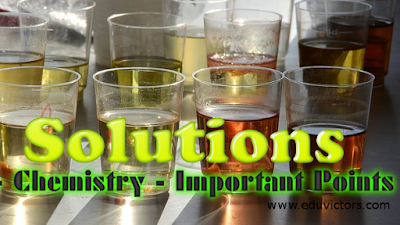Solutions -Very Short Questions and Answers

Q1: What is Van’t Hoff factor ?

Answer: It is the ratio of normal molecular mass to observed molecular mass. It is denoted
as i.
i = normal molecular mass/observed molecular mass
= no. of particles after association or dissociation/no. of particles before

Q2: What is the Van’t Hoff factor in K₄[Fe(CN)₆] and BaCl₂ ?

Q3: Why the molecular mass becomes abnormal ?

Answer: It is due to association or dissociation of solute in given solvent.

Q4: Define molarity. How it is related with normality ?

Answer: N = M ✕ Basicity or acidity

Q5: How molarity is related with percentage and density of solution ?

Answer:  M = P ✕ d ✕ 10/M.M₂

Q6: What role does the molecular interaction play in the solution of alcohol and water?

Answer: Positive deviation from ideal behaviour.

Q7: What is van’t Hoff factor ? How is it related with :
(a) degree of dissociation
(b) degree of association

(a) α = i – 1/n – 1
(b) α = i – 1/1/n – 1

Q8: Why NaCl is used to clear snow from roads?

Answer: It lowers freezing point of water.

Q9: Why the boiling point of solution is higher than pure liquid?

Answer: Due to lowering in vapour pressure.

Q10: Out of 1 M and 1 m aqueous solution which is more concentrated ?

Answer: 1 M as density of water is 1 gm/ml.

Q11: Henry law constant for two gases are 21.5 and 49.5 atm, which gas is more soluble?

Answer: Kₕ is inversely proportional to solubility.

Q12: Why water cannot be completely separated from aqueous solution of ethyl alcohol?

Answer: Due to formation of azeotrope at (95.4%).

Q13: What is the molarity of pure water ?

Q14: Give one use of reverse osmosis

Q15: Give an example of a compound in which hydrogen bonding results in the formation of a dimer.

Q16: What is the difference between electrolyte and non-electrolyte?

Answer: An electrolyte is a substance that, when dissolved in a solvent or melted, conducts an electrical current. A nonelectrolyte does not conduct a current when dissolved.

Q17: Give four examples of colligative properties:

vapor pressure lowering
freezing-point depression
boiling-point elevation
osmotic pressure

Q18: Define Henry's law.

Answer: The solubility of gaseous state in a liquid solvent at constant temperature is directly proportional to the pressure of gaseous state.